Slope and Y-Intercept From the Graph1.

Uphill or downhill?
Positive or negative slope?
What is the slope (m)?
What is the y-intercept (b)?
What is the equation?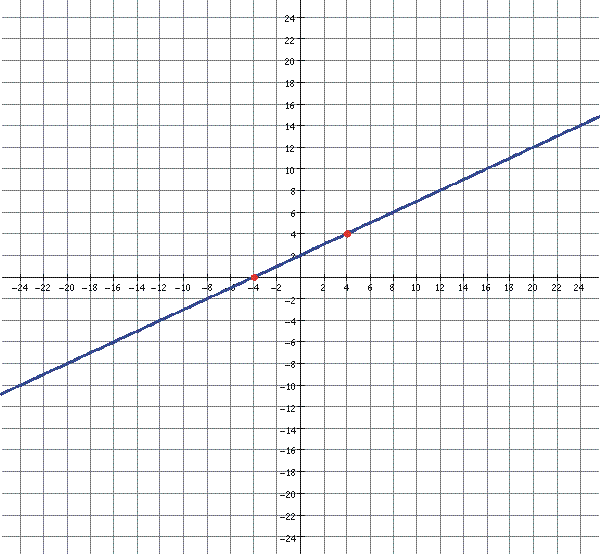2.

Uphill or downhill?
Positive or negative slope?
What is the slope (m)?
What is the y-intercept (b)?
What is the equation?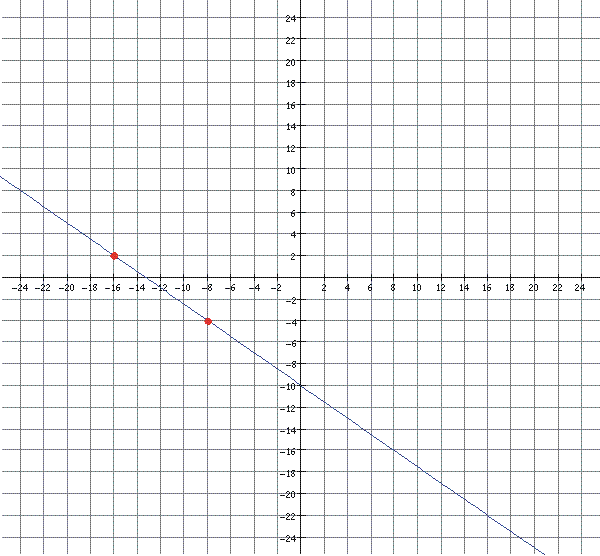3.

Uphill or downhill?
Positive or negative slope?
What is the slope (m)?
What is the y-intercept (b)?
What is the equation?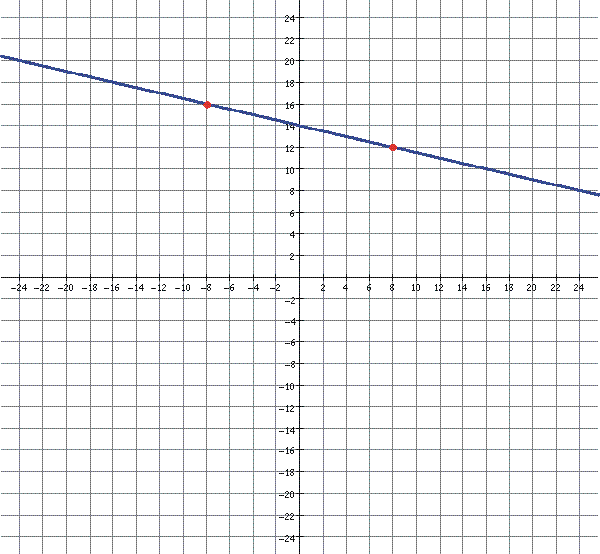4.

Uphill or downhill?
Positive or negative slope?
What is the slope (m)?
What is the y-intercept (b)?
What is the equation?5.

Uphill or downhill?
Positive or negative slope?
What is the slope (m)?
What is the y-intercept (b)?
What is the equation?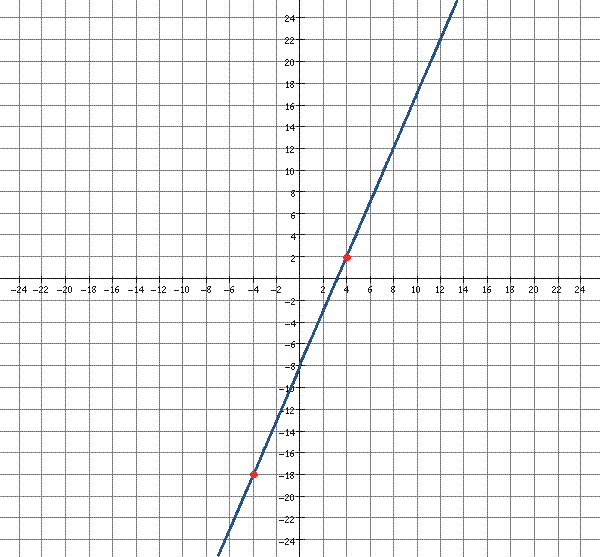6.

Uphill or downhill?
Positive or negative slope?
What is the slope (m)?
What is the y-intercept (b)?
What is the equation?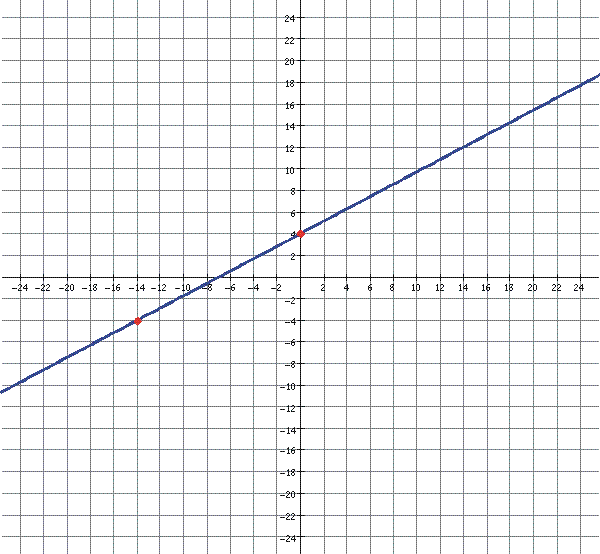7.

Uphill or downhill?
Positive or negative slope?
What is the slope (m)?
What is the y-intercept (b)?
What is the equation?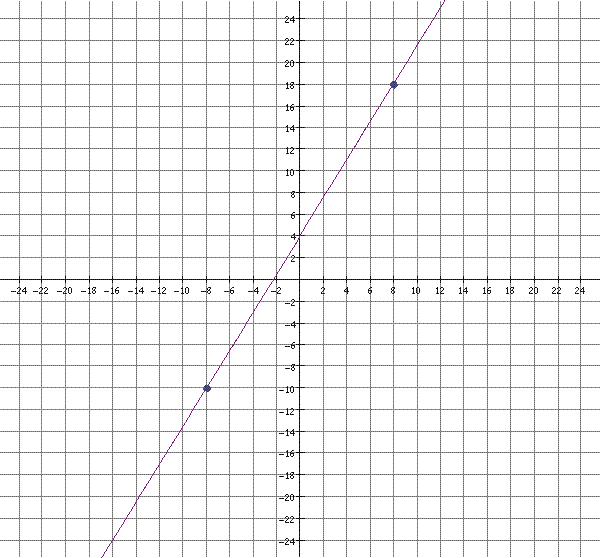8.

Uphill or downhill?
Positive or negative slope?
What is the slope (m)?
What is the y-intercept (b)?
What is the equation?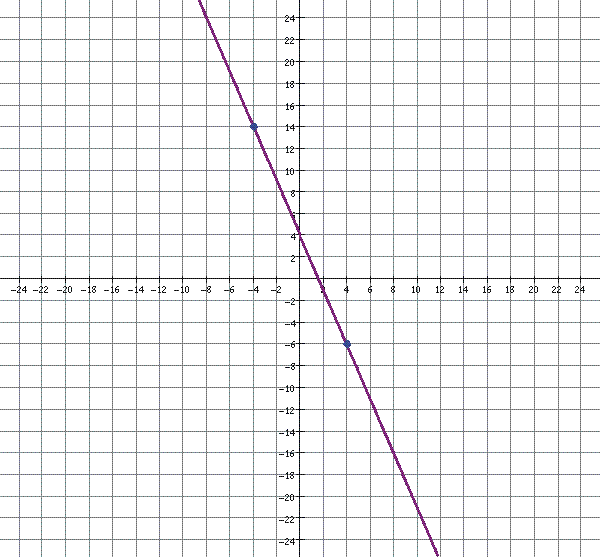9.

Uphill or downhill?
Positive or negative slope?
What is the slope (m)?
What is the y-intercept (b)?
What is the equation?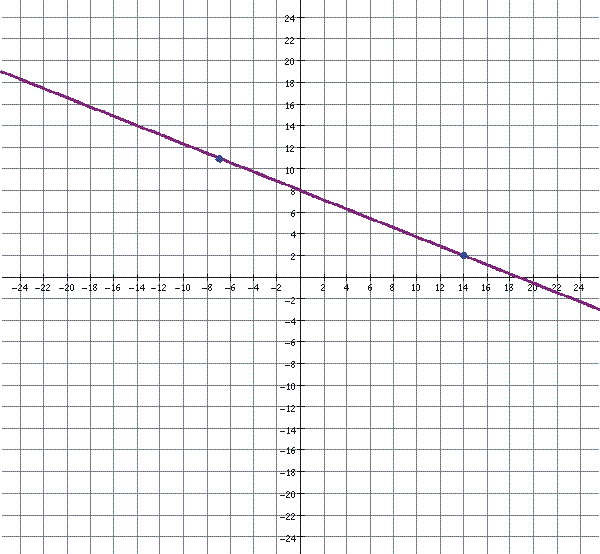10.

Uphill or downhill?
Positive or negative slope?
What is the slope (m)?
What is the y-intercept (b)?
What is the equation?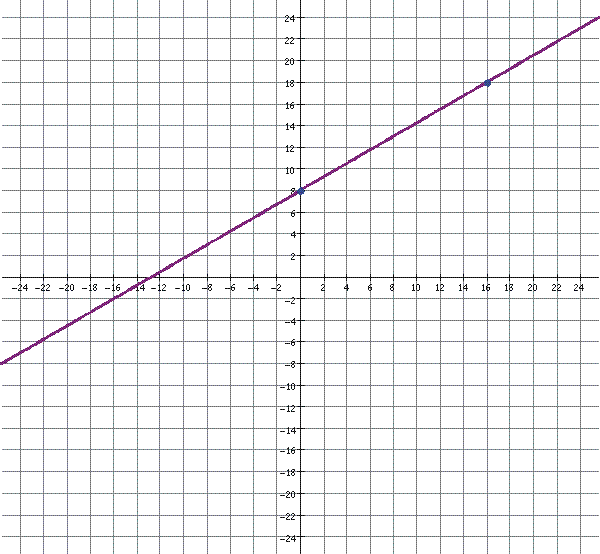11.

Uphill or downhill?
Positive or negative slope?
What is the slope (m)?
What is the y-intercept (b)?
What is the equation?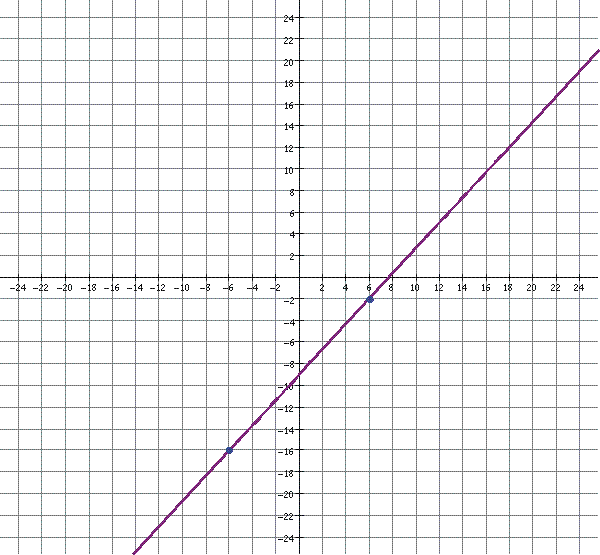12.

Uphill or downhill?
Positive or negative slope?
What is the slope (m)?
What is the y-intercept (b)?
What is the equation?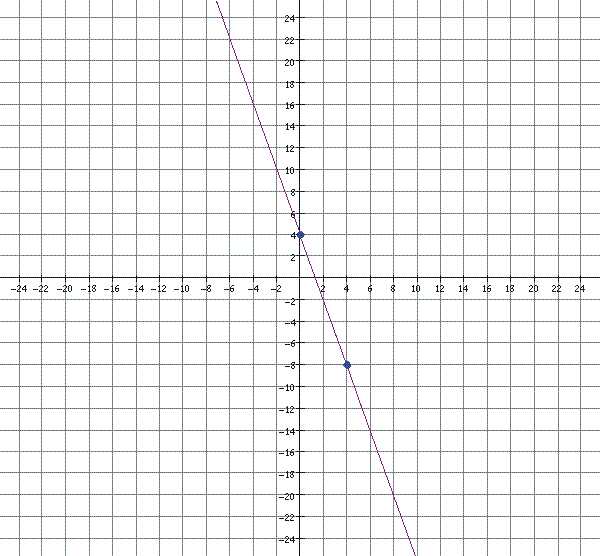13.

Uphill or downhill?
Positive or negative slope?
What is the slope (m)?
What is the y-intercept (b)?
What is the equation?14.

Uphill or downhill?
Positive or negative slope?
What is the slope (m)?
What is the y-intercept (b)?
What is the equation?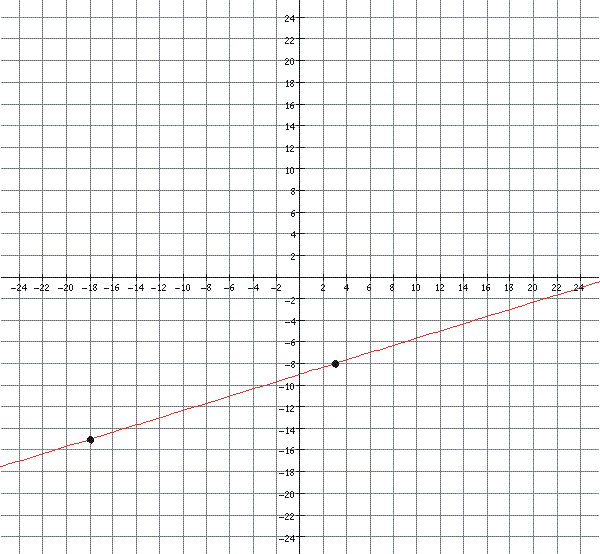15.

Uphill or downhill?
Positive or negative slope?
What is the slope (m)?
What is the y-intercept (b)?
What is the equation?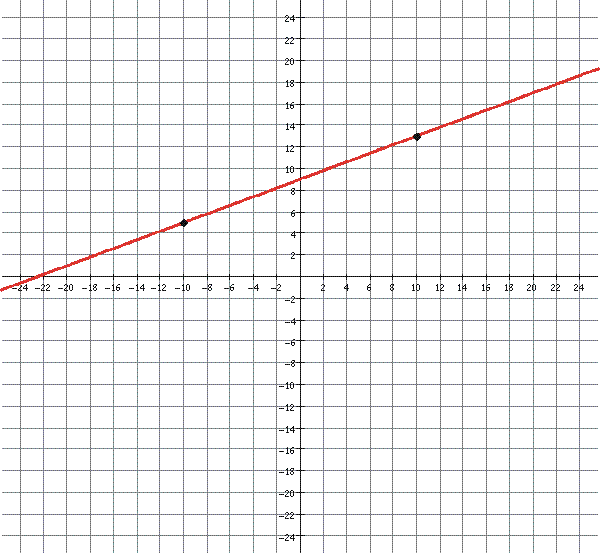16.

Uphill or downhill?
Positive or negative slope?
What is the slope (m)?
What is the y-intercept (b)?
What is the equation?17.

Uphill or downhill?
Positive or negative slope?
What is the slope (m)?
What is the y-intercept (b)?
What is the equation?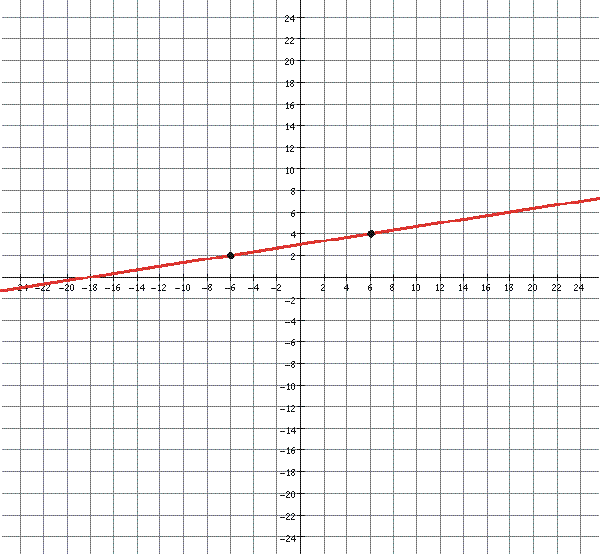18.

Uphill or downhill?
Positive or negative slope?
What is the slope (m)?
What is the y-intercept (b)?
What is the equation?19.

Uphill or downhill?
Positive or negative slope?
What is the slope (m)?
What is the y-intercept (b)?
What is the equation?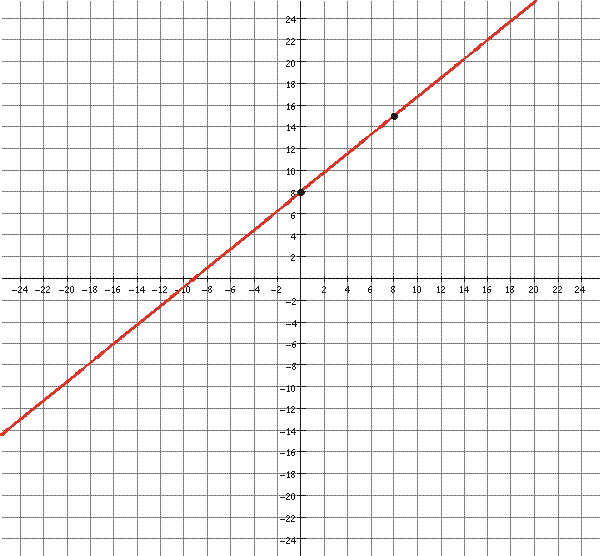20.

Uphill or downhill?
Positive or negative slope?
What is the slope (m)?
What is the y-intercept (b)?
What is the equation?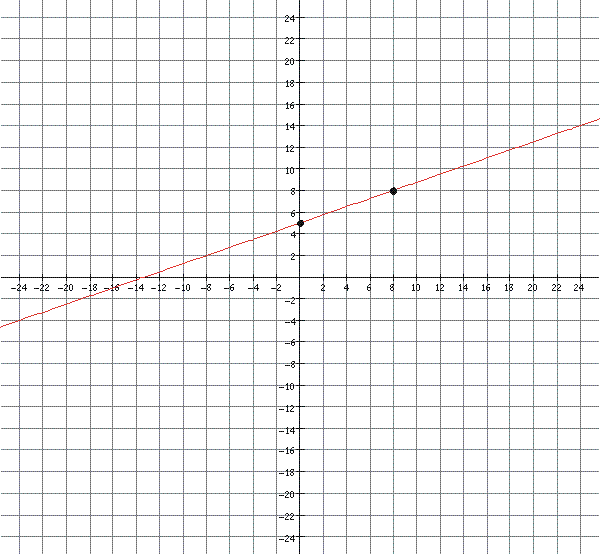21.

Uphill or downhill?
Positive or negative slope?
What is the slope (m)?
What is the y-intercept (b)?
What is the equation?